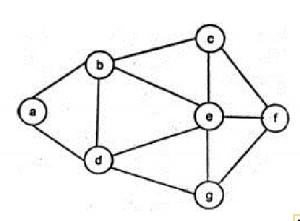# GATE | Gate IT 2008 | Question 45

Consider the following sequence of nodes for the undirected graph given below.

a b e f d g c
a b e f c g d
a d g e b c f
a d b c g e f
A Depth First Search (DFS) is started at node a. The nodes are listed in the order they are first visited. Which all of the above is (are) possible output(s)?(A) 1 and 3 only
(B) 2 and 3 only
(C) 2, 3 and 4 only
(D) 1, 2, and 3